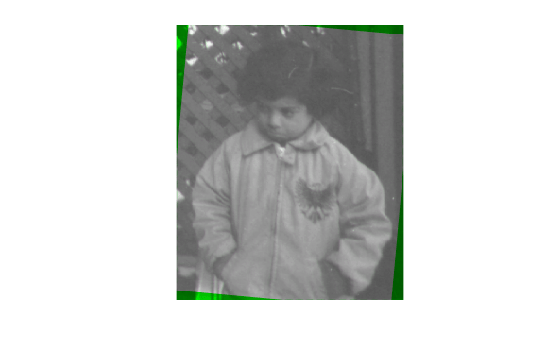# MeanSquares

Mean square error metric configuration

## Description

A `MeanSquares` object describes a mean square error metric configuration that you pass to the function `imregister` to solve image registration problems.

## Creation

You can create a `MeanSquares` object using the following methods:

• `imregconfig` — Returns a `MeanSquares` object paired with an appropriate optimizer for registering monomodal images

• Entering

`metric = registration.metric.MeanSquares;`
on the command line creates a `MeanSquares` object

## Examples

collapse all

Create a `MeanSquares` object and use it to register two images with similar brightness and contrast.

Read the reference image and create an unregistered copy.

```fixed = imread('pout.tif'); moving = imrotate(fixed,5,'bilinear','crop');```

View the misaligned images.

`imshowpair(fixed,moving,'Scaling','joint');`Create the metric configuration object suitable for registering monomodal images.

`metric = registration.metric.MeanSquares`
```metric = registration.metric.MeanSquares This class has no properties. ```

Create the optimizer configuration object.

`optimizer = registration.optimizer.RegularStepGradientDescent;`

Modify the optimizer configuration to get more precision.

```optimizer.MaximumIterations = 300; optimizer.MinimumStepLength = 5e-4;```

Perform the registration.

`movingRegistered = imregister(moving,fixed,'rigid',optimizer,metric);`

View the registered images.

`imshowpair(fixed, movingRegistered,'Scaling','joint');`## Tips

• The mean squares metric is an element-wise difference between two input images. The ideal value is zero. You can examine the computed values of mean square error if you enable `'DisplayOptimization'` when you call `imregister`. For example, ```movingRegistered = imregister(moving,fixed,'rigid',optimizer,metric,'DisplayOptimization',true);```

## Algorithms

The mean squares image similarity metric is computed by squaring the difference of corresponding pixels in each image and taking the mean of the squared differences.

## Version History

Introduced in R2012a

expand all